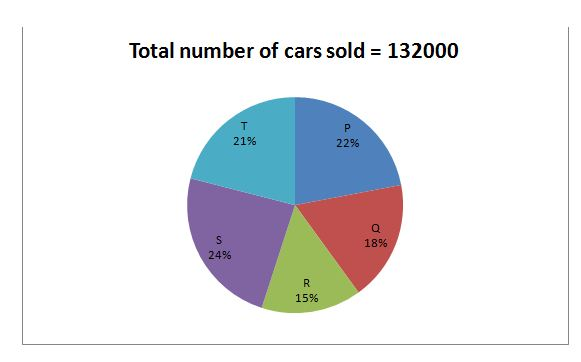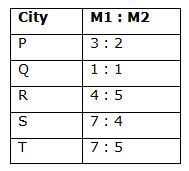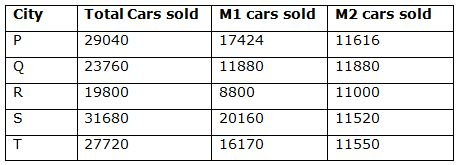# IBPS SO / NIACL AO Prelims – Quantitative Aptitude Questions Day- 62

Dear Readers, Bank Exam Race for the Year 2018 is already started, To enrich your preparation here we have providing new series of Practice Questions on Quantitative Aptitude – Section. Candidates those who are preparing for IBPS SO / NIACL AO Prelims 2018 Exams can practice these questions daily and make your preparation effective.

[WpProQuiz 4704]

Directions (Q. 1 – 5): What value should come in place of question mark (?) in the following number series?

1) 52, 26.5, 27.5, 42.75, ?

a) 58.5

b) 66.25

c) 72.75

d) 87.5

e) 92.25

2) 27, 54, 162, 648, ?

a) 1786

b) 2890

c) 3240

d) 2154

e) 3278

3) 137, 137, 142, 157, ? , 477

a) 195

b) 222

c) 298

d) 326

e) 387

4) 207, 225, 245, 268, 296, 331, ?

a) 388

b) 400

c) 377

d) 436

e) 392

5) 796, 399, 136, 39, ?

a) 14.8

b) 11.2

c) 15.6

d) 13.4

e) 12.8

Directions (Q. 6 – 10) Study the following information carefully and answer the given questions:

The following pie chart shows percentage distribution of total number of cars sold in five different cities and the table shows the ratio of two different model cars sold in those cities.6) Find the total number of M2 cars sold in all the five cities together?

a) 51788

b) 53445

c) 49113

d) 57566

e) None of these

7) Total number of M1 cars sold in City P, R and T together is approximately what percentage more/less than the total number of M2 cars sold in City Q, S and T together?

a) 21 % less

b) 32 % more

c) 21 % more

d) 32 % less

e) None of these

8) Which city has sold highest number of M1 cars?

a) City S

b) City Q

c) City R

d) City P

e) City T

9) Find the difference between the total number of M2 cars sold in City P and Q together to that of total number of M1 cars sold in City S and T together?

a) 11678

b) 12834

c) 13116

d) 14552

e) None of these

10) Find the ratio between the total number of cars sold in City P, R and T together to that of total number of cars sold in City Q, R and S together?

a) 526: 421

b) 613: 517

c) 638: 627

d) 597: 491

e) None of these

Directions (1-5):

The pattern is, *0.5 + 0.5, *1 + 1, *1.5 + 1.5, *2 + 2,…

The pattern is, *2, *3, *4, *5,…

The pattern is, +12 -1, +22 + 1, +42 – 1, +82 + 1, +162 – 1,…

The difference of difference is, 2, 3, 5, 7, 11,… (Prime no’s)

The pattern is, ÷ 2 + 1, ÷ 3 + 3, ÷ 4 + 5, ÷ 5 + 7,..

Directions (6-10):Total number of M2 cars sold in all the five cities together,

= > 11616 + 11880 + 11000 + 11520 + 11550

= > 57566

Total number of M1 cars sold in City P, R and T together

= > 17424 + 8800 + 16170

= > 42394

The total number of M2 cars sold in City Q, S and T together

= > 11880 + 11520 + 11550

= > 34950

Required % = [(42394 – 34950)/34950]*100

= > (7444/34950)*100 = 21.29 % = 21 % more

In city P, M1 cars = 17424

In city Q, M1 cars = 11880

In city R, M1 cars = 8800

In city S, M1 cars = 20160

In city T, M1 cars = 16170

So, City S sold highest number of M1 cars.

The total number of M2 cars sold in City P, Q together

= > 11616 + 11880 = 23496

The total number of M1 cars sold in City S, T together

= > 20160 + 16170 = 36330

Required difference = 36330 – 23496 = 12834

The total number of cars sold in City P, R and T together

= > 29040 + 19800 + 27720 = 76560

The total number of cars sold in City Q, R and S together

= > 23760 + 19800 + 31680 = 75240

Required ratio = 76560: 75240 = 638: 627